Almost forgot!

First order goes with FREE EXTRA - plagiarism report. How cool is that?

ORDER NOWThanks, but I don’t like free stuff

# Sample on Statistics Project

Often students come to us saying, “Write my essay, please, it’s so boring I’m afraid of dislocating my jaw!” However, the sample below shows how any topic can be made exciting – with a pinch of creativity.

Statistics Project

### Introduction

In this paper we will discuss and describe how the basic tools of statistics analysis and probability theory may be applied to a real world problem. The data given is a breakdown of all 426 goals made by Lionel Messi during his football career. There are 90 observations of the data; each represents the number of goals during a game played by L. Messi. Our goal is to perform multiple steps of statistical analysis: we build frequency distribution characteristics – frequency tables and histograms, pie charts, elements of descriptive statistics (measures of central tendency and measures of variability). In addition to the descriptive part, we also perform a hypothesis testing part to compare mean values between two groups of the data.

Body

First of all, it should be mentioned that on 90th position of our sample we have the sum of all goals made by Lionel Messi from 90th game and since today. That’s why this indicator may bias the results of our procedures as it doesn’t represent the number of goals in a single game. We would like to exclude this observation from the sample and proceed with only the rest.

For the first part of our research, we begin with a frequency distribution of the data using first 67 observations. The number of goals in this subsample varies from 0 to 12. That’s why we decide to divide the data by 6 classes: 0-2,3-5, 6-8, 9-11, 12. The width of classes is 2. A frequency table has the following form:

 Class Frequency Percentage 0-2 19 28.36% 3-5 33 49.25% 6-8 12 17.91% 9-11 2 2.99% 12 1 1.49% Total 67 100%

To present the data distribution in the most suitable form, we construct frequency histogram with a normal curve. This step allows us to compare the distribution of the given variable with a normal distribution: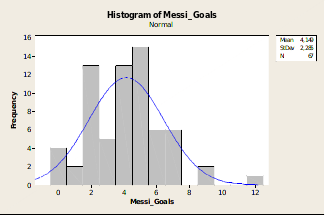The next step of the research is to complete a Pie Chart. We do not use classes in this case, just for each value of goals during a game we calculate percentage and represent it on a Pie Chart: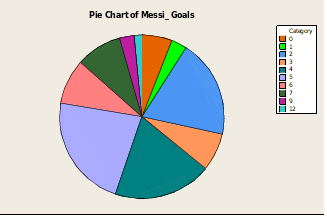As the next step of the project we have to calculate the basic measured of descriptive statistics: measures of central tendency and measures of variability. Everything what is required, is included in the table below:

Descriptive Statistics: Messi_Goals

N for

Variable Mean TrMean StDev Variance Median Mode Mode

Messi_Goals 4,149 4,066 2,285 5,220 4,000 5 15

Also, midrange is: 6

Weighted mean is: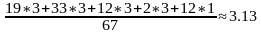The obtained from descriptive statistics information about the distribution allows us to do the following conclusions. The measures of the “middle” are different. We can see that Mean is 4.149, mode is 5, median is 4, trimmed mean is 4.066, weighted mean is 3.13.

This difference caused by the fact that the distribution is not normal; it is a little positively skewed. Besides, there are few outliers of the data, such as 12 goals in 1 game (an unusual observation). For a skewed data, the best measure of the middle is median value.

For the second part of the paper we have to divide the data on 2 groups. The first group is 67 observations from the very beginning and the second group is the remaining data. Since we do not know the population standard deviation (we have only summary statistics for 90+ games), we use Student’s t-test instead of z-test.

Null hypothesis: there is no significant difference in goal numbers between two groups

Alternative hypothesis: there is a significant difference in goal numbers between two groups.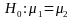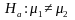Level of significance is 5%: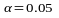Run t-test:

Two-Sample T-Test and CI: Messi_Goals_1; Messi_Goals_2

Two-sample T for Messi_Goals_1 vs Messi_Goals_2

N Mean StDev SE Mean

Messi_Goals_1 67 4,15 2,28 0,28

Messi_Goals_2 23 6,39 4,84 1,0

Difference = mu (Messi_Goals_1) – mu (Messi_Goals_2)

Estimate for difference: -2,24

95% CI for difference: (-4,40; -0,09)

T-Test of difference = 0 (vs not =): T-Value = -2,14 P-Value = 0,042 DF = 25

We can see that p-value is 0.042 which is < 0.05, thus we reject the null hypothesis. We support the claim that there is a significant difference in goal numbers between two groups (at 5% level of significance). This might be because Lionel Messi performed better in his latest games compared to the beginning of his career.

## Our services

Prices are dropping. Now only \$9.97 per page. Limited offer. Hurry up! PricesChat now
Call us (Toll Free)